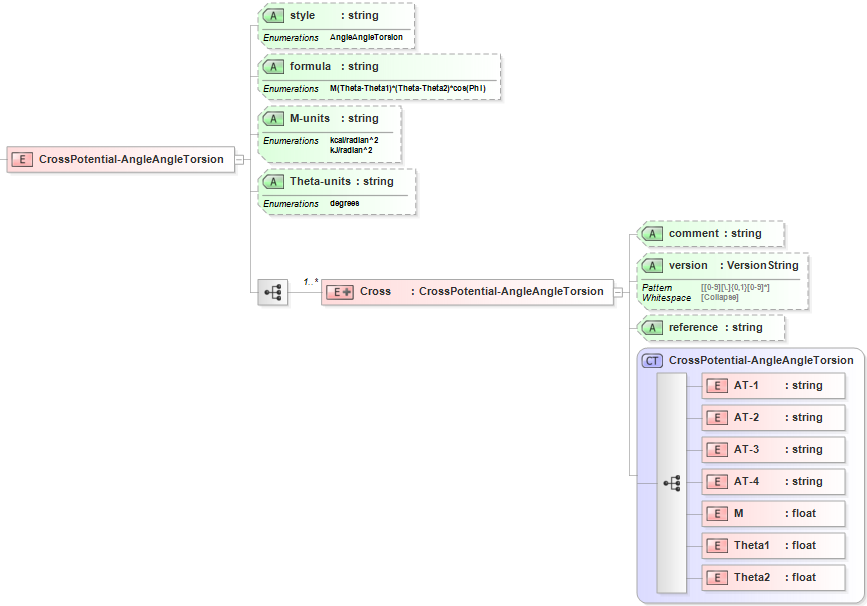7.8. Cross: Angle-Angle-Torsion¶

7.8.1. Functional Form¶

The Angle-Angle-Torsion Cross Potential has the functional form:

$$E={{M}_{ijkl}}\left( {{\theta }_{ijk}}-{{\theta }_{1,ijk}} \right)\left( {{\theta }_{jkl}}-{{\theta }_{2,jkl}} \right)\cos \left( {{\phi }_{ijkl}} \right)$$

This term is part of the Class2 Dihedral Potential style.

The force-field parameters for this potential and units are given by:

 Equation Symbol Parameter Definition Units $$M_{ijkl}$$ Bond coefficient for atoms [i,j,k,l] energy $${\theta}_{1,ijk}$$ Equilibrium angle for atoms [i,j,k] degrees $${\theta}_{2,jkl}$$ Equilibrium angle for atoms [j,k,l] degrees

7.8.2. XML Schema¶

The XML schema for the Angle-Angle-Torsion Cross Potential has the following representation (design mode representation using Liquid XML Studio):The relationship between the equation symbols and XML schema notations are given by:

 Parameter Definition Equation Symbol Schema Notation Atom type of atom [i] $$i$$ AT-1 Atom type of atom [j] $$j$$ AT-2 Atom type of atom [k] $$k$$ AT-3 Atom type of atom [l] $$l$$ AT-4 Bond coefficient for atoms [i,j,k,l] $$M_{ijkl}$$ M Equilibrium angle for atoms [i,j,k] $${\theta}_{1,ijk}$$ Theta1 Equilibrium angle for atoms [j,k,l] $${\theta}_{2,jkl}$$ Theta2

The general attributes (describing the entire data set) are given by:

 General Attributes Cardinality Value/Definition style Fixed AngleAngleTorsion formula Fixed M(Theta-Theta1)*(Theta-Theta2)*cos(Phi) M-units Required Enumerations specified in schema Theta-units Required Enumerations specified in schema

The specific attributes (attached to each set of parameters) are given by:

 Specific Attributes Cardinality Value/Definition comment Optional Comment attached to parameter set version Optional Version number of parameter set reference Optional Reference attached to parameter set

Note that an XML document will be rejected from being entered into the WebFF database if a required attribute is left unspecified.